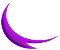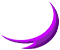## panel dimensions calculator

First lets look at how a panel is defined by the design of the lampshade.Each tier in the lampshade is defined by 3 measurements - the ones I use here are the diameter of the lampshade at the top of the panel and the bottom of the panel and the vertical height of the panel's tier.

By diameter I mean the diameter of a circle which passes through the internal corners of the lampshade - if you have an even number of sides, this will equal the corner to opposite corner measurement.

If you want an extremely precise result, you will need to make an allowance for the thickness of the glass (usually ~3mm) - a typical approach is to subtract twice the thickness of the glass (say 6mm) from the diameter dimensions.These dimensions are easy to determine from a profile or side-view (elevation) drawing of the lampshade.

However - if you need a precise result (within a millimetre or similar) with the size of the lampshade you need to be aware of the size disparity between the design dimensions and the resulting constructed size.

The calculations assume that the diameters supplied are the internal dimensions - shown by the red arrow. Most people seem to measure lamshade by their external dimensions - shown by the blue arrow. There is a difference, if it is important to you then you are better off using the external diameter measurements.Here are the dimensions which define an individual panel, once you know these you can draw an accurate outline for the panel cartoon or cutting drawing.Here is how you specify the dimensions of your lampshade for this calculator, when you click the calculate button a new window should open with a list of the panel dimensions for each tier of the lampshade.

The nature of lampshades means that the bottom diameter of any tier is the same measurement as the top diameter for the tier below (except for the final or bottom-most tier naturally).

This calculator handles a maximum of 6 tiers at a time. If your lampshade has more tiers - calculate the first six tiers then return here and enter the values for the next 6 tiers and then calculate them and so on.

The units are for your convenience only - the calculations are identical for imperial and metric units.⇒These values must be entered to get useful results. number of sides (between 3 and 100)unit of measurement you are using how many decimals do you want? what are your measurements? inner diameter outer diametertop-most diametertop tier vertical heightbottom of top tier diametersecond tier vertical height bottom of second tier diameter third tier vertical height bottom of third tier diameter fourth tier vertical height bottom of fourth tier diameter fifth tier vertical height bottom of fifth tier diameter sixth tier vertical height bottom of sixth tier diameter
Many Blessingsreturn to top of this pagebackground courtesy Clachan Chalanais (the Standing Stones of Callanish):||:
-->